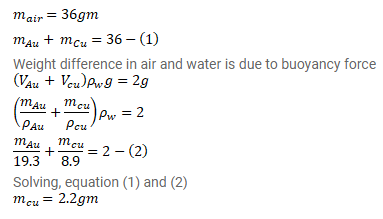# An ornament weighing 36g in air,

Question:

An ornament weighing $36 \mathrm{~g}$ in air, weighs only $34 \mathrm{~g}$ in water. Assuming that some copper is mixed with gold to prepare the ornament, find the amount of copper in it. Specific gravity of gold is $19.3$ and that of copper is $8.9$

Solution: